# Multiple Comparisons With Unequal Sample Sizes

## David C. Howell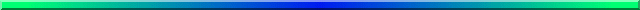Because the question that prompted this note referred specifically to the Newman-Keuls test, I will answer with respect to that test. However the approach generalizes to any of the multiple comparison procedures that are based on a t or q statistic.

It is important to keep in mind that the Newman-Keuls, the Tukey, and a number of other tests use the same arithmetic, but only differ in the critical value that they require for significance. The Tukey uses q with r set at the number of means in the sample. The Newman-Keuls uses q with r set at the number of means of which the two in question are the largest and smallest. Other tests behave similarly. So whatever I say below for the Newman-Keuls can be readily translated to other tests.

The solution for doing a Newman-Keuls test with unequal sample sizes is basically the same solution you would use for a variety of post-hoc procedures. Most of the post-hoc tests involve some sort of t test or Studentized range test. As such, they contain a standard error of the form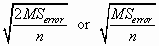The former is used with a t test, and the latter with a Studentized range-based test (such as the Newman-Keuls or Tukey tests.)

The problem with either of these formulae is that they assume that you have a constant sample size. If you have different sample sizes, you need to replace "n" with "ni" and "nj."

There are two ways to do this. The simpler, known as the Tukey-Kramer approach, is to assume that the populations have equal variances, and therefore to continue to use MSerror as our variance estimate. Thus the formulae would be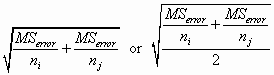again, using the first if you have a t test and the second if you have a Studentized range test.

Notice that these formulae, and those that follow, assume that you carry out separate calculations of the error term for each pair of samples. That is because ni and nj will change as you change the two groups you are comparing. This is a pain in the neck, but you don't have much choice.

Using this approach, you can calculate either t or q, and evaluate them against the t or Studentized range tables.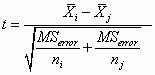and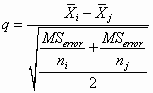In both cases the degrees of freedom would equal the degrees of freedom for MSerror.

If you want to calculate a critical width (Wr) instead of a test statistic like t or q, you can simply multiply the appropriate error term by the critical value of t on dferror or by the critical value of q for r and dferror.

Games and Howell (1976) (no relation) carried this one step further by allowing for heterogeneous sample variances, as well as unequal sample sizes. They proposed an error term of the form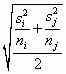Here again you will be required to calculate a separate error term for each pair of samples.

Games and Howell went a bit further, recognizing that with this error term the degrees of freedom need to be adjusted. Their adjustment goes back to the adjustments proposed by Welch and by Satterthwaite, and can be written as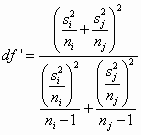This, too, must obviously be computed for each pair of samples.

Again you can form the t or q test statistic by replacing the standard (common) error term with the individualized error term above, and using df' instead of dferror for your degrees of freedom.

As I say elsewhere, if the sample sizes are nearly equal, you can save a great deal of time by using the more traditional formulae and substituting the harmonic mean of the sample sizes. I do not recommend this if you have heterogeneous variances.dch